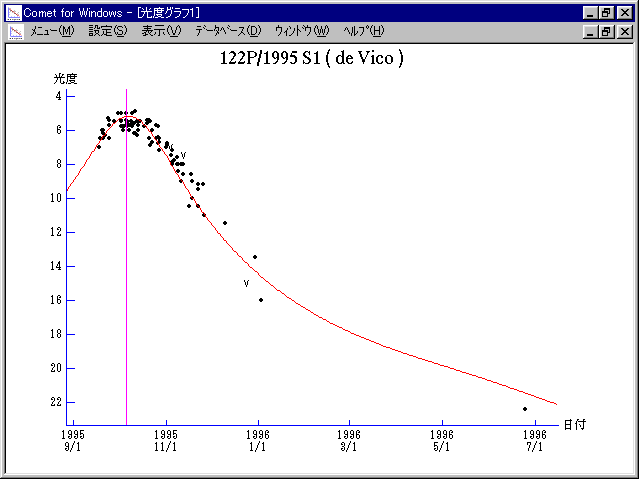# \$B%G!&%t%#%3WB@1(B

122P/de Vico (1995)###\$B%W%m%U%#!<%k(B

 \$BId9f(B 122P/1995 S1 \$B:FH/8+F|(B 1995\$BG/(B9\$B7n(B17\$BF|(B \$B:FH/8+8wEY(B 7.0\$BEy(B \$B:FH/8+ \$BCfBO8c(B (\$B7'K\(B), \$BEDCf@/L@(B (\$BJ!Eg(B), D. E. Machholz (Colfax, CA), \$B4XJY(B (\$B9bCN(B)

###\$B50F;MWAG(B

```   The following improved orbital elements,  by Kenji Muraoka, are
from 211 observations 1995 Sept. 18 to 1996 June 25, perturbations
by 9 Planets, Moon and 5 minor planets were taken into account.
The mean residual is +/- 0.97 arc seconds.

Epoch  =  1995 Oct. 10.0  TT       JDT = 2450000.5
T  =  1995 Oct.  6.02381       +/- 0.00002 (m.e.) TT
Peri. =   12.97682                +/- 0.00004
Node  =   79.61807                +/- 0.00003   (2000.0)
Incl. =   85.39119                +/- 0.00002
q  =    0.6588922              +/- 0.0000001 AU
e  =    0.9627360              +/- 0.0000014
a  =   17.6817347              +/- 0.0000364 AU
n  =    0.01325613             +/- 0.00000004
P  =   74.351                  +/- 0.0002297  years
```

###\$B@1?^(B1995\$BG/(B 5\$B7n(B 3\$BF|!A(B1995\$BG/(B10\$B7n(B20\$BF|(B1995\$BG/(B 9\$B7n(B20\$BF|!A(B1996\$BG/(B 5\$B7n(B 7\$BF|(B

###\$B8wEYJQ2=(B

```        m1 = 8.5 + 5 log\$B&\$(B + 18.0 log r
```##### \$B50F;MWAG\$OB<2,7r<#;a\$N7W;;\$K\$h\$k\$b\$N\$G\$9!#(B \$B@1?^\$O(B StellaNavigator Ver.2.0 for Windows (\$B%"%9%H%m%"!<%D(B \$BJTCx(B / \$B%"%9%-!<=PHG6I4)(B) \$B\$G:n@.\$7\$?\$b\$N\$G\$9!#(B \$B8wEY%0%i%U\$O(BComet for Windows\$B\$G:n@.\$7\$?\$b\$N\$G\$9!#(B